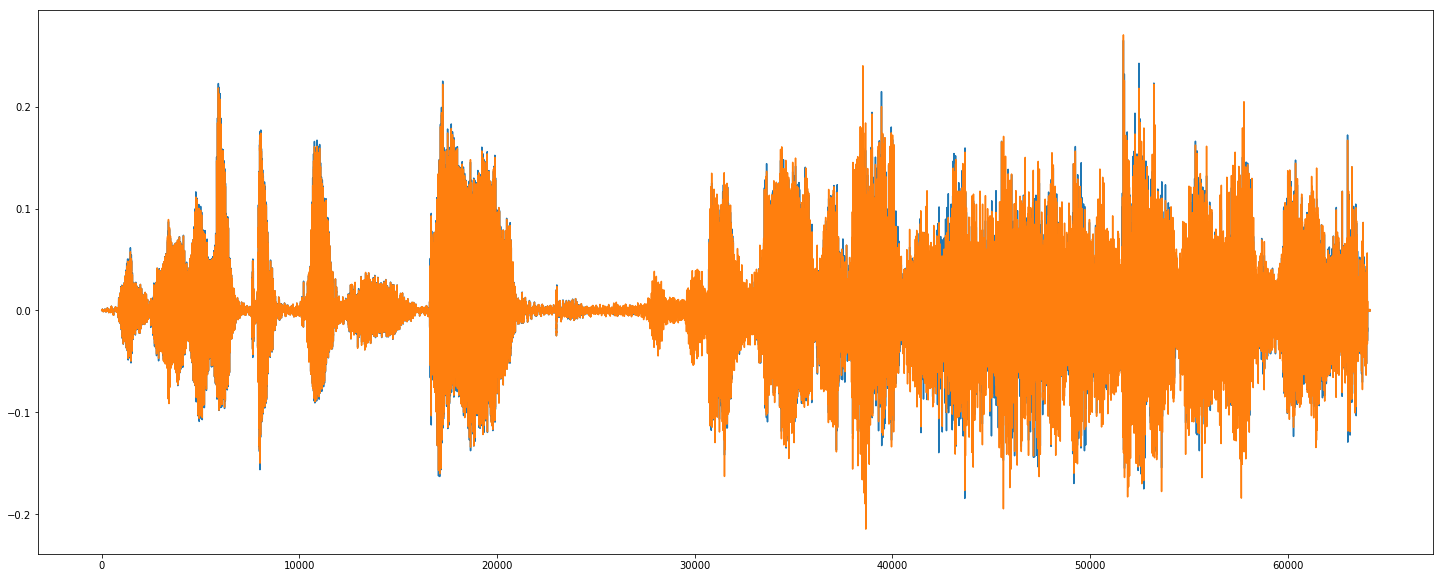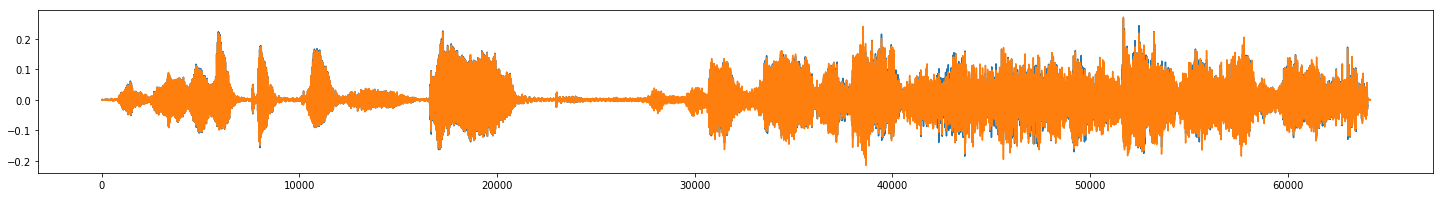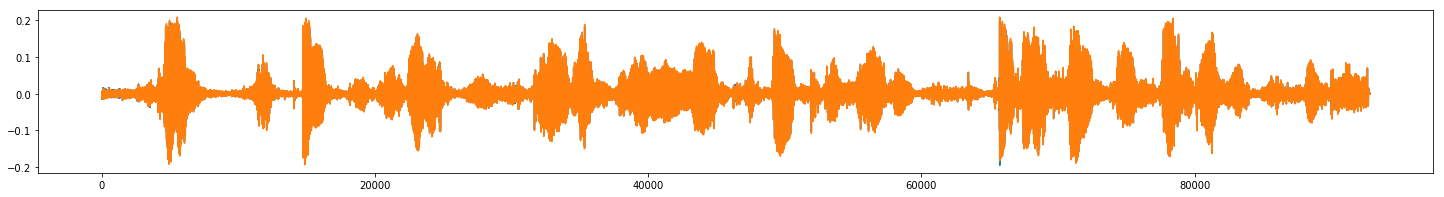# PIPELINE¶

1. Explore the data.
2. Remove outliers, clean the data.
3. Get Data labels
5. Build the Model.
6. Train the Model.
7. Analyse model performance.
8. Save the model and add logs.

#  PRELIMINARY¶

In :
# Making the code cells wider. Feel free to skip/disable.

from IPython.core.display import display, HTML
display(HTML('<style>/.container { width:97% !important; }</style>'))

In :
'''IMPORTS'''

import os
import numpy as np
import pandas as pd
import matplotlib.pyplot as plt

from tqdm import tqdm as tqdm
import torch
import torch.nn as nn
import torchvision.transforms as transforms
import torchaudio
import pickle
from sklearn.metrics import confusion_matrix

# Use GPU is available
device = torch.device("cuda:0")
torch.cuda.is_available()

Out:
False
• It is assumed that the code files are in the folder 'emotion'.
• Thus the five train folders should be in emotion/meld/train".
• Similarly for validation data.
In :
'''SETTING ROOT DIRECTORIES'''

train_folder_path = "emotion/meld/train"
valid_folder_path = "emotion/meld/val"
emotion_list = np.array(["disgust", "fear", "happy", "neutral", "sad"])
emotion_labels = np.array(["DIS", "FEA", "HAP", "NEU", "SAD"])


We store the paths of all files in the training(and validation) set as numpy arrays. The dataloader then preprocesses them, and then they are fed into the Model for training.

In :
'''COLLECTING TRAIN FILE PATHS'''

train_paths = []

for emotion in emotion_list:
folder_path = os.path.join(train_folder_path, emotion)

for file_name in os.listdir(folder_path):
file_path = os.path.join(folder_path, file_name)
train_paths.append(file_path)

# Convert into numpy array.
train_paths = np.array(train_paths)

In :
'''COLLECTING VALID FILE PATHS'''

valid_paths = []

for emotion in emotion_list:
folder_path = os.path.join(valid_folder_path, emotion)

for file_name in os.listdir(folder_path):
file_path = os.path.join(folder_path, file_name)
valid_paths.append(file_path)

# Convert into numpy array.
valid_paths = np.array(valid_paths)

In :
print(len(train_paths))
print(len(valid_paths))

7354
830


Sort all the files in order to avoid different indices when dealing with outliers later.

In [ ]:
train_paths.sort()
valid_paths.sort()

In [ ]:


In [ ]:



#  EXPLORATORY DATA ANALYSIS¶

Plotting a sinlge audio waveform.

In :
'''PLOTTING A SINGLE WAVEFORM'''

f = train_paths

print("Shape of waveform: {}".format(waveform.size()))
print("Sample rate of waveform: {}".format(sample_rate))

plt.figure(figsize=[25,10])
plt.plot(waveform.t())
plt.plot()

Shape of waveform: torch.Size([2, 64171])
Sample rate of waveform: 16000

Out:
[]Plotting multiple audio waveforms.

In :
'''SAMPLE AUDIO FILES AND WAVEFORMS'''

from IPython.display import Audio, display

for i in range(5):

print(train_paths[i])
print(t.shape)

# Display interactive output.
display(Audio(train_paths[i]))

# Plot the waveform.
plt.figure(figsize=[25,3])
plt.plot(t.t())
plt.show()

emotion/meld/train/disgust/MEL_dia1000_utt0_negative_DIS.wav
torch.Size([2, 64171])emotion/meld/train/disgust/MEL_dia1000_utt1_negative_DIS.wav
torch.Size([2, 92843])emotion/meld/train/disgust/MEL_dia1005_utt13_negative_DIS.wav
torch.Size([2, 9216])